#### You may also like### DOTS Division

Take any pair of two digit numbers x=ab and y=cd where, without loss of generality, ab > cd . Form two 4 digit numbers r=abcd and s=cdab and calculate: {r^2 - s^2} /{x^2 - y^2}.### Novemberish

a) A four digit number (in base 10) aabb is a perfect square. Discuss ways of systematically finding this number. (b) Prove that 11^{10}-1 is divisible by 100.### Latin Numbers

Can you create a Latin Square from multiples of a six digit number?

# Number Rules - OK

##### Age 14 to 16Challenge Level

We received a lot of excellent solutions to this problem which showed that a lot of people put in a lot of time and effort. We have tried to mention and quote everyone, but there is not enough space to include all of the good work that we received. Well done!

1. Two consecutive numbers add to give an odd number
Miraya from Heckmondwike Grammar School in the UK and Le Duc Anh Ken from British Vietnamese International School in Vietnam tried some examples. Here is Le Duc Anh Ken's work: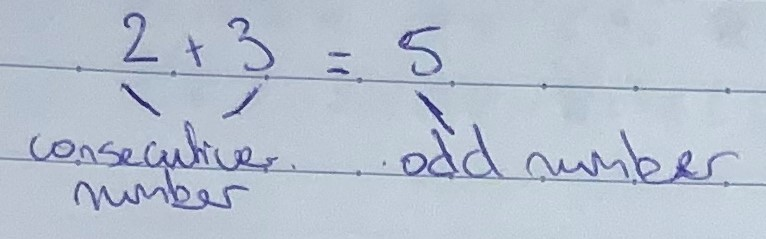And Miraya's examples:

1+2=3    2+3=5    3+4=7    4+5=9    5+6=11.....

Nayanika from The Tiffin Girls' School and Olivia from Dulwich College Beijing exlained why the answer is always odd. Olivia wrote:

In two consecutive numbers there is always one odd and one even, so if we take 1 away from the odd one we get two even numbers.
Two even numbers added together is bound to be another even number, so when you add the 1 we took from the odd number before to this even number, you get an odd number.

Eric from Lakeridge in the USA, Rin from Wren Academy in England, Lareina from Dulwich College Beijing in China, Seyon from Wilson's School in the UK and Sunhari expressed the numbers algebraically. Seyon wrote:

Keep the first consecutive number as $x$. The next shall be $x+1$. So the total of the two is: $2x+1$.

Mahdi from Mahatma Gandhi International School in India and Muhammad C from Bolton School Boys' Division showed that this is an odd number. This is Mahdi's work: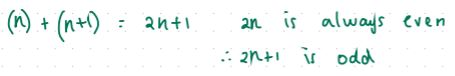And this is Muhammad C's work:Sanika from PSBBMS, OMR in India thought about two different situations:

Case 1 (when the first number is even):
$n_1 = 2k$
$\Rightarrow n_2 = 2k+1$
$n_1+n_2 = 4k+1$
Since all multiples of $4$ are even, the number next to a multiple of $4$ will be odd. So case 1 will give an odd number.

Case 2 (when the first number is odd)
$n_1 = 2k+1$
$\Rightarrow n_2 = 2k+1 +1 =2k+2$
$n_1+n_2=4k+3 = 4(k+1)-1$
The number before an even number is always odd. Since all multiples of $4$ are even, case 2 will always give an odd result.

2. The product of two consecutive numbers is even
Le Duc Anh Ken sent in this example: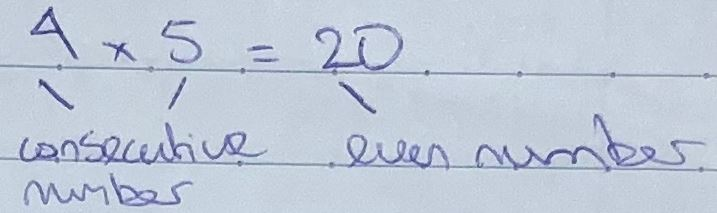Miraya gave these examples:

2$\times$3 = 6   3$\times$4 = 12   5$x\times$6 = 30  6$\times$7 = 42    7$\times$8 = 56  ....

Eric, Lareina, Muhammad C and Mahdi wrote why this is true. Eric wrote:

Numbers go odd-even-odd-even... and even times anything is even.

Olivia explained why that is true:

Product means the answer you get when you multiply something and multiplication is just repeated addition. Repeatedly adding even numbers would always equal even, so repeatedly adding the even number (for the amount of times equal to the odd number, or any number really) could only equal even.

Nayanika used the last digits: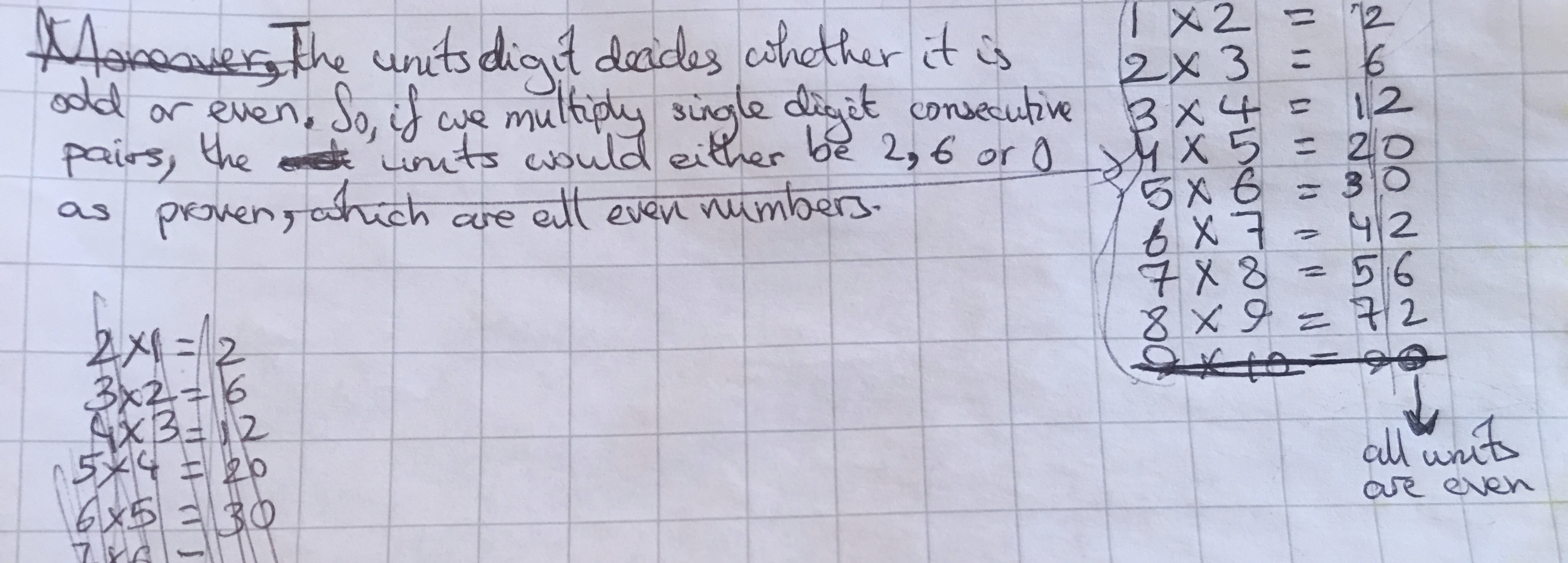Sunhari, Rin and Sanika used algebra to represent the two consecutive numbers. This is Sanika's work:

Case 1 (when the first number is even):
$n_1 = 2k$
$\Rightarrow n_2 = 2k+1$
$n_1n_2 = 2(k)(2k+1)$
As the product of the numbers contains $2$ as one of its factors, case one will give an even result.

Case 2 (when the first number is odd)
$n_1 = 2k+1$
$\Rightarrow n_2 = 2k+1 +1 =2(k+1)$
$n_1n_2=2(k+1)(2k+1)$
As the product of the numbers contains $2$ as one of its factors, case two will give an even result.

3. The sum of four consecutive numbers is never a multiple of $4$
Here are Miraya's examples:

1+2+3+4=10    2+3+4+5=14   25+26+27+28=106....

Nayanika noticed that the examples can form a sequence. She also proved that the sum of five consecutive numbers is always a multiple of 5!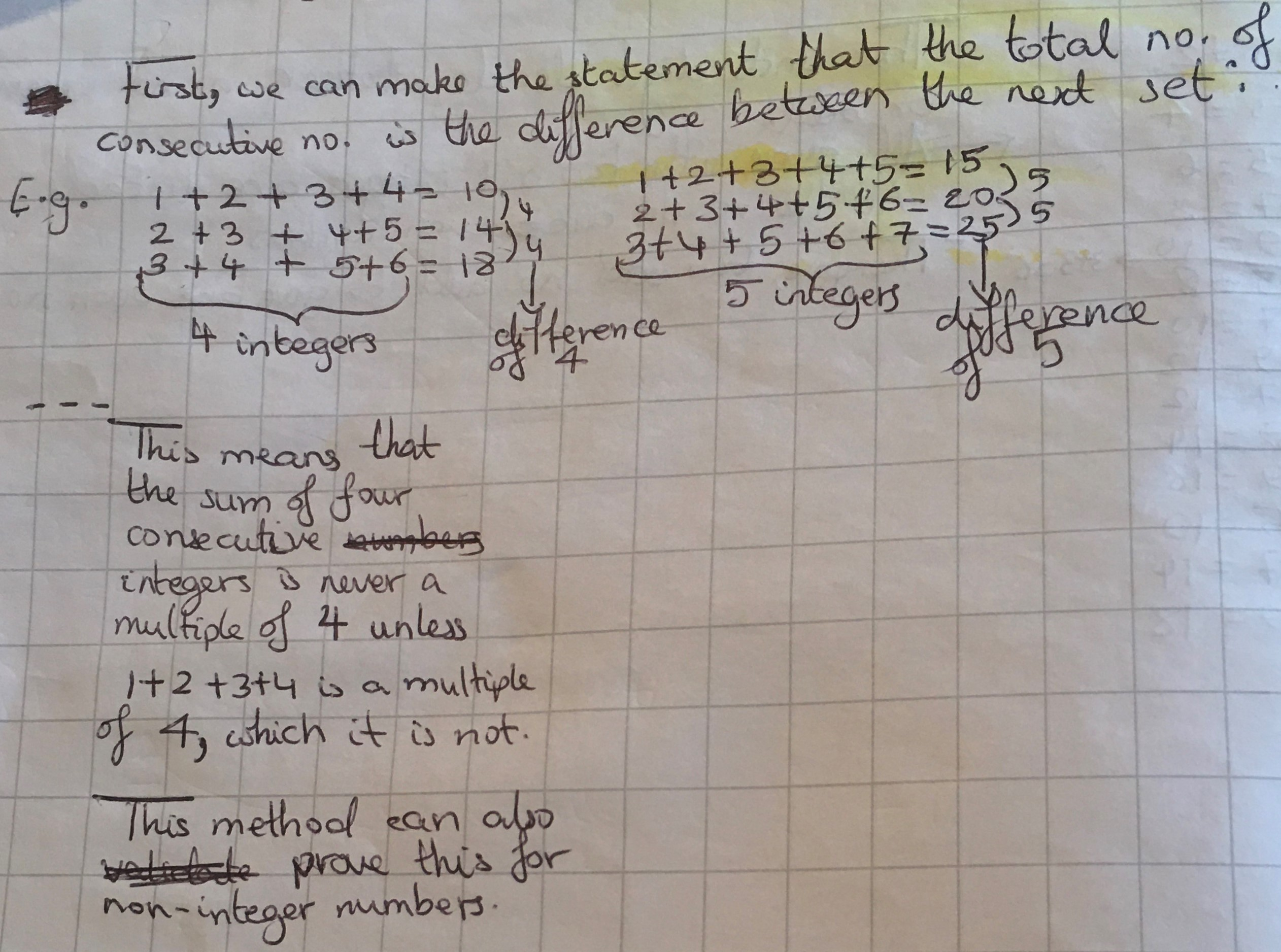Eric and Sunhari wrote the numbers algebraically. Sunhari wrote:

$n + (n+1) + (n+2) + (n+3)$
$= n + n +1 + n + 2 + n +3$
$= 4n + 6$

Miraya, Rin and Mahdi used remainders to show that $4n+6$ is never a multiple of $4.$ Rin wrote:

$4n+6=4(n+1)+2$ ($n$ is an integer)
The sum will always give a remainder of $2$ when dividing by $4$
$\therefore$ it will never be a multiple of $4$.

Muhammad C showed it in a different way: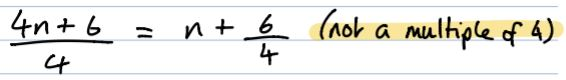Lareina and Olivia showed it in yet another way. This is Lareina's work: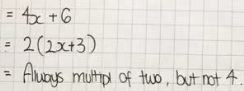Sanika considered four different cases! Click to see Sanika's work.

Case 1: $n_1 \text{ mod }4=0$
$n_1=4k$       $n_2=4k+1$       $n_3=4k+2$        $n_4=4k+3$
$n_1+n_2+n_3+n_4=16k+4+2=4(4k+1)+2$
Case 1 gives us a remainder of $2$ when divided by $4$ and is consequently not divisible by $4.$

Case 2: $n_1 \text{ mod }4=1$
$n_1=4k+1$      $n_2=4k+2$       $n_3=4k+3$        $n_4=4(k+1)$
$n_1+n_2+n_3+n_4=16k+8+2=4(4k+2)+2$
Case 2 also gives us a remainder of $2$ when divided by $4.$

Case 3: $n_1 \text{ mod }4=2$
$n_1=4k+2$      $n_2=4k+3$       $n_3=4(k+1)$        $n_4=4(k+1)+1$
$n_1+n_2+n_3+n_4=16k+12+2=4(4k+3)+2$
Case 3 also gives us a remainder of $2$ when divided by $4.$

Case 4: $n_1 \text{ mod }4=3$
$n_1=4k+3 n_2=4(k+1) n_3=4(k+1)+1 n4=4(k+1)+2$
$n_1+n_2+n_3+n_4=16k+12+2=4(4k+4)+2$

4. Two odd numbers add to give an even number
Miraya and Le Duc Anh Ken tried some examples. These are Miraya's examples:

3+5=8    5+7=12 ...  99+101=200 ...

Nayanika used the last digits idea again: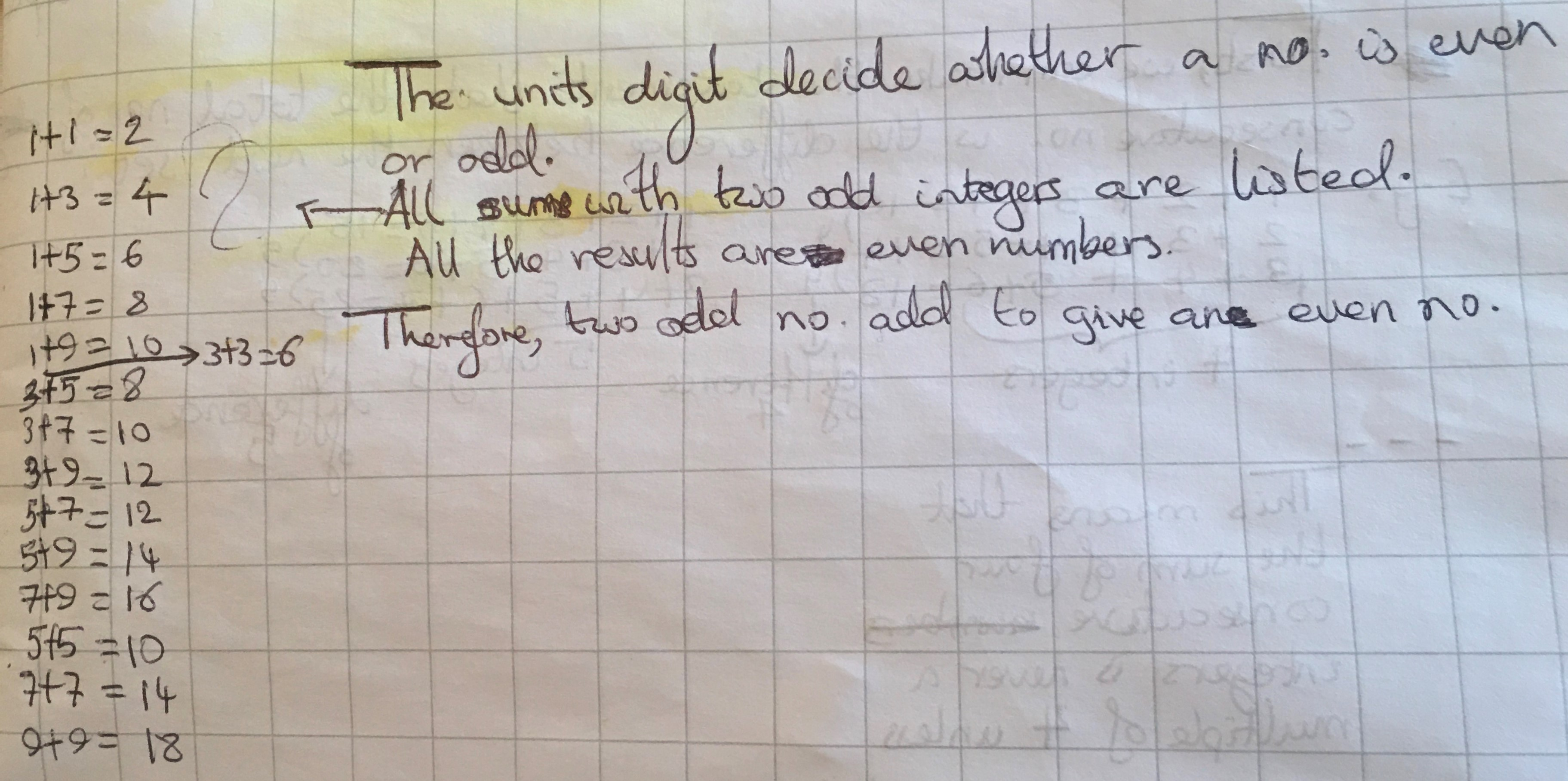Olivia explained intuitively why it works:

Taking 1 away from an odd number gives an even number so if you take 1 away from both the odd numbers, you get two even numbers which you add together to make another even number. The even number plus the 2 we took away previously (one from each odd number) would still be even because even plus even equals even.

Muhammad C, Rin, Sunhari and Mahdi used algebra to prove it. This is Muhammad C's work: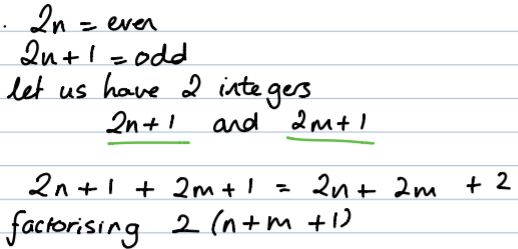Miraya also used algebra. Miraya wrote:

The expressions $2n-1$ and $2n+1$ represent odd numbers, as an odd number is one less and one more than an
even number. $2n-1+2n+1= 4n$, which is ALWAYS EVEN.

But $2n-1$ and $2n+1$ can't represent any two odd numbers. They represent two odd numbers which are two apart, like 5 and 7, 7 and 9, 9 and 11, 683 and 685... So Miraya has proved that the sum of two consecutive odd numbers is always even.

5. The pattern given continues forever
Lareina described the pattern in words: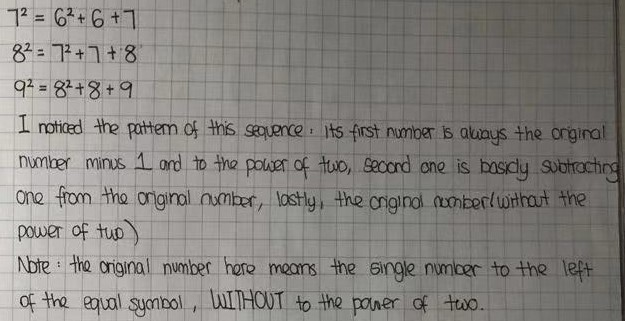Eric showed how the pattern works using an image. A bit of explanation has been added to Eric's diagram.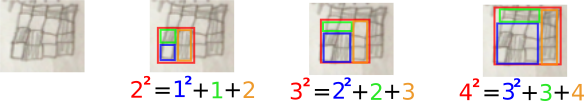Miraya, Muhammad C and Sanhari used algebra with $n$ and $n-1$. This is Olivia's work:

$n^2=(n-1)^2+(n-1)+n$
$n^2=n^2+-2n+n+n$
$n^2=n^2$
The first line I wrote is just the rule written out algebraically, the second line is after I expanded the brackets and the third line is after I simplified the second line because $-2n+n+n$ just crosses itself out. That leaves $n^2=n^2$ which proves the case.

Nayanika, Rin and Mahdi used algebra slightly differently. This is Mahdi's work: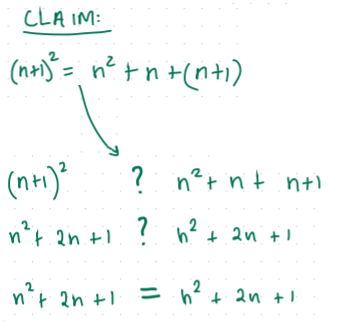Notice that Mahdi used a ?, not an =, until he had proved that the two sides were equal. Some people started with the statement they wanted to prove, and finished with "$n^2=n^2$" or "$0=0$". This is like starting from a statement you think is true and working to a statement you know is true (like "$0=0$"). You should really start from something you know is true and work to the statement you think is true - then you will have proved it!

6. Squaring an odd number always gives an odd number
Eric and Nayanika used the final digits. Eric wrote:

Units digit is $1, 3, 5, 7, 9$.

$1^2, 3^2, 5^2, 7^2, 9^2$ is odd.

Miraya, Muhammad C, Rin, Olivia and Mahdi used algebra. Miraya wrote:

Firstly, an algebraic expression of an odd number should be identified, such as $2n+1$ or $2n-1.$ Doing this would also indicate the knowledge that $2n$ is always an even number.

This should then be written out as $(2n+1)(2n+1)$.
Multiplying these two expressions together gives us $4n^2 +2n + 2n +1$, or $4n^2 +4n +1.$

In order to prove this is odd, we can simply take a factor of $2$ out of the first two terms to leave us with $2(2n^2+2n) +1.$
If we now refer to $2n^2+2n$ as $x$, we can rewrite this equation as $2x +1$, which is the same algebraic expression we used to identify a number as odd. We can therefore state that the square of any odd number is always odd too.

7. If a square number is multiplied by a square number the product is a square number
Here are some examples from Miraya and Le Duc Anh Ken:

$2^2\times3^2 = 4\times 9 = 36 = 6^2$
$4\times4=16$ SQUARE NUMBER
$9\times81=729$  SQUARE NUMBER
$9\times16=144$ SQUARE NUMBER

Eric, Rin, Sunhari and Mahdi proved it using algebra. This is Rin's work:

The product of two square numbers can be written as:
$n^2m^2=(nm)^2$  [$n$ and $m$ are integers]
$nm$ is an integer
$\therefore$ The product of two square numbers is always a square

Some people wrote the two square numbers as $n^2$ and $n^2$. But $n^2$ and $n^2$ is the same square number twice! Rin used $m^2$ and $n^2$ to represent two different square numbers.

### Final Challenge - your own rules

Le Duc Anh Ken wrote that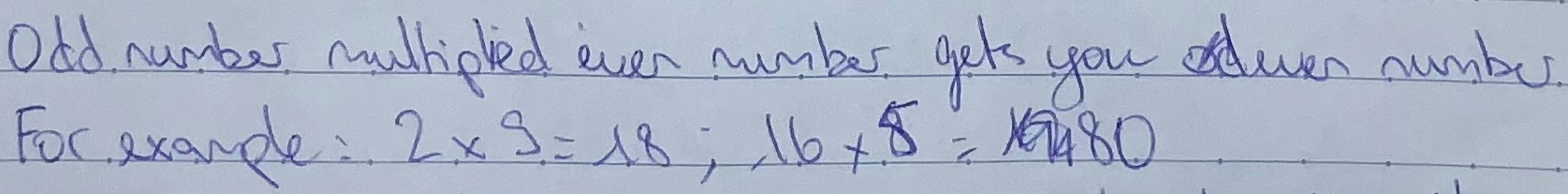In fact, some people used (and proved) this rule for rule number 2 above.

Sunhari sent in and proved two rules:

I) Two odd numbers always have an odd product.
$(2n+1)(2x+1)$
$= 4nx + 2n + 2x + 1$
$= 2(2nx+n+x)+1$

II) Three odd numbers sum up to an odd.
$2n + 1 + 2x + 1 + 2y + 1$
$=3(2(n+x+y) + 1))$ [Note this should be $2(n+x+y)+3$]
We know $2(n+x+y)+1$ is an odd number and (I) states that for two odd numbers, the product is an odd number.
[Note that $2(n+x+y)+3$ = $(2(n+x+y)+1)+2$ so $2(n+x+y)+3$ is the next odd number afer $2(n+x+y)+1$]

Rin found and proved another rule:

Prove that $n(n-1)(2n-1)$ is always a multiple of $6$.

First, $n$ and $n-1$ are consecutive; one of these must be even.
$\therefore$ If $n(n-1)(2n-1)$ is a multiple of $3$, the statement is true.

$n$ can always be written as either $3k, 3k+1$ or $3k+2$    [$k$ is an integer]

i.    when $n=3k$
$n(n-1)(2n-1)$
$=3k(3k-1)(6k-1)$
As $k(3k-1)(6k-1)$ is an integer, $n(n-1)(2n-1)$ is always a multiple of $3$

ii.    when $n=3k+1$
$n(n-1)(2n-1)$
$=(3k+1)3k(6k+1)$
As $(3k+1)k(6k+1)$ is an integer, $n(n-1)(2n-1)$ is always a multiple of $3$

iii.    when $n=3k+2$
$n(n-1)(2n-1)$
$=(3k+2)(3k+1)(6k+3)$
$=(3k+2)(3k+1)(2k+1)3$
As $(3k+2)(3k+1)(2k+1)$ is an integer, $n(n-1)(2n-1)$ is always a multiple of $3$

$\therefore$ For any integer $n$, $n(n-1)(2n-1)$ is a multiple of $3$ and even. Hence it is always a multiple of $6.$

GG from Wilson's School in England sent in this rule. Can you work out how it works?

Pick a number that ends with 5. Now, forget the 5 and think of your new number, e.g.  25 becomes 2.
Now find the next ascending consecutive number, e.g. 2 and 3. Multiply those 2 numbers together, e.g. 2$\times$3=6.
tick a 25 onto the end, e.g. 625.
Now remember the number you started with, e.g. 25. The number that resulted after sticking 25 onto the end is the square of the number you first thought of.

Sanika sent in this number rule and proof. The proof uses a formula which has not been mentioned above.

Rule: Sum of the first n odd numbers is equivalent to $n^2$

Proof: Sum of an arithmetic sequence $= \frac{n}2(2a+d(n-1))$
$a=1$ (as we are starting the series of odd numbers with $1$)
$d=2$ (common difference between odd numbers)
$\Rightarrow s_n=\frac{2n}2(1+(n-1))\\ \Rightarrow s_n=n(n)=n^2$